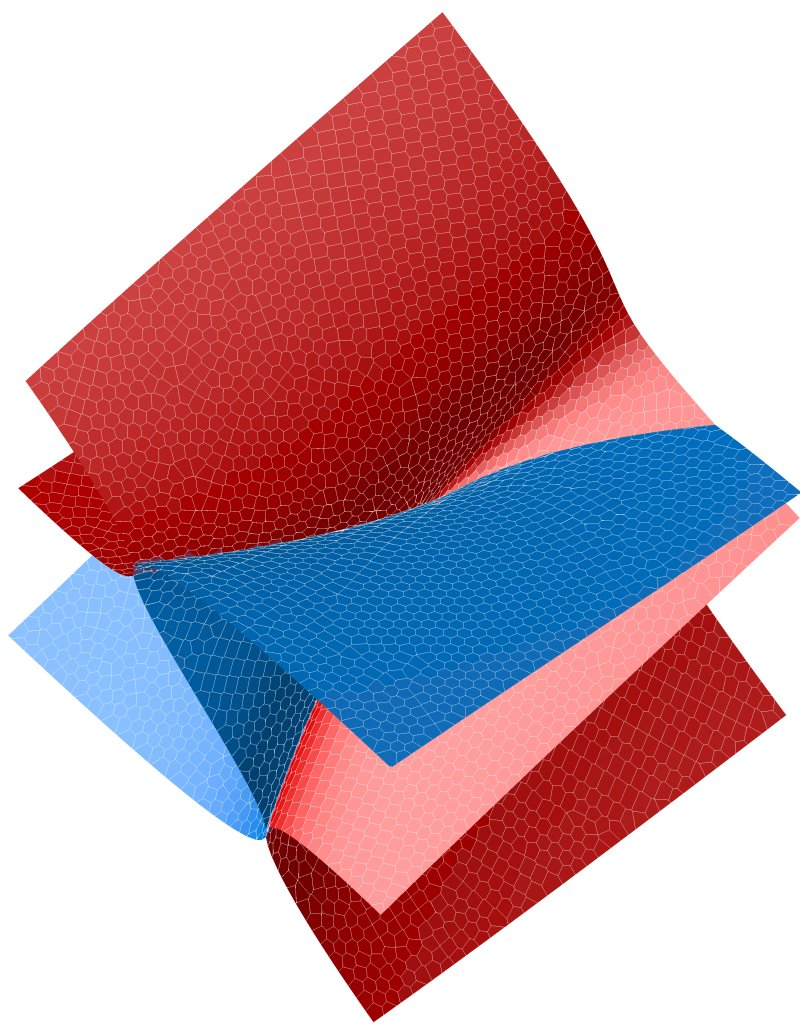# Michael E Hendersongen_feedback_link(leftr, rightr);

## contact information

Principal Research Staff Member, Applied Mathematics
Thomas J. Watson Research Center, Yorktown Heights, NY USA

### Professional Associations

Professional Associations:  ACM  |  IEEE   |  Society for Industrial and Applied Mathematics  |  Tau Beta Pi - The Engineering Honor Society

## Examples of the Multifario Continuation Algorithm

This new version of the implicit surface algorithm uses the interior of hyperspheres as the domains of charts covering the manifold, instead of ellipses. This allows the boundary of the union of the balls covering the manifold to be computed as the union of intersections of hypersphere and a convex polyhedron.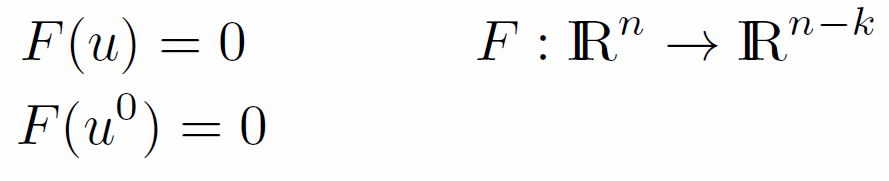These examples were all generated using the same algorithm!

### Circle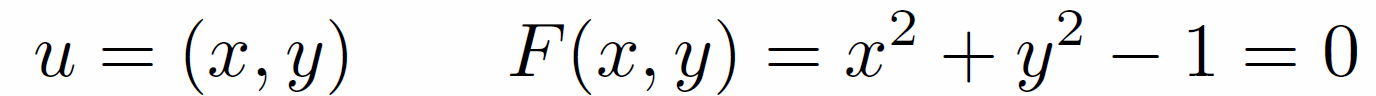A 1-manifold embedded in 2-space: n=2, k=1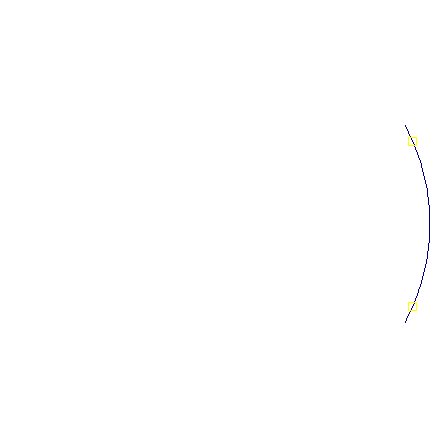The circle is computed, starting from an initial point, by covering a small neighborhood of the initial point with a mapping of an interval from the tangent line to the circle. The projection of an endpoint of the interval is then used to select a new point on the circle.

As each new point is added, the intervals around each point are shortened so that the projection of the intervals do not overlap (approximate). Endpoints of the original intervals are then eligible for use to generate a new point. The shortening is done by projecting one interval into the tangent lines at nearby points. The algorithm terminates when there are no longer any endpoints that an be selected.

The result is a set of points on the circle, and a set of edges connecting the centers of intersecting intervals.

### Plane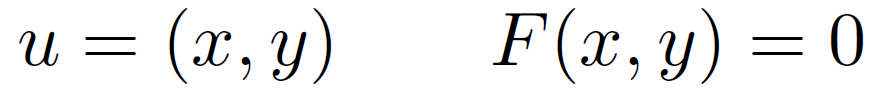A 2-manifold embedded in 2-space: n=2, k=2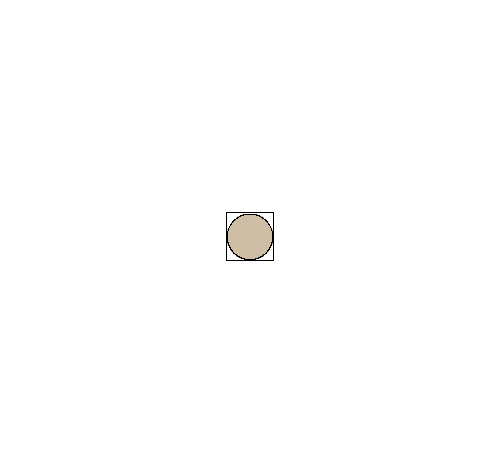In computing the plane the intervals in the tangent line (above) become circles. Circles are trimmed by removing half-planes. This creates polygons, beginning with squares slightly larger than the circle (red edges). If all of the vertices of the polygon lie inside the circle there is no boundary of the circle left in the collection of circles. If a vertex lies outside the circle, a line joing the center of the circle to the vertex crosses the circle at a boundary point (yellow).

The blue edges are the dual of the polygons. This is roughly analogous to the Delaunay triangulation of the points.

In this example the circles are all the same size. The algorithm does not require this.

Anyone know of an implementation of the coloring that the four color theorem assures us exists?

### Sphere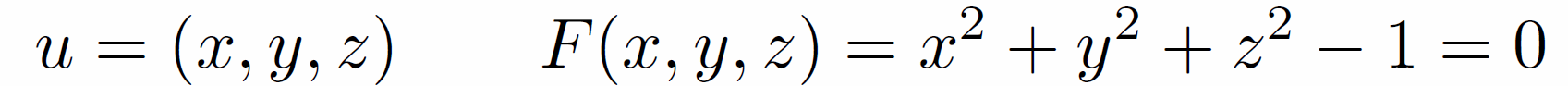A 2-manifold embedded in 3-space: n=3, k=2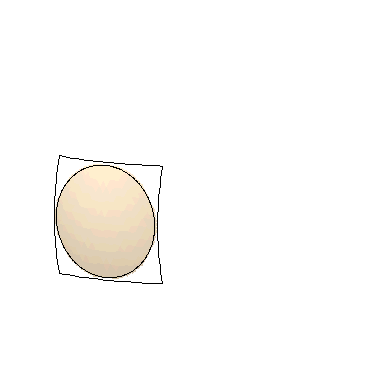The Sphere is similar to the computation of the plane, except that the circles and polygons live in the tangent plane. The intersections are done by projecting neigboring circles into the tangent plane and approximating them by circles.

### Torus

A 2-manifold embedded in 3-space: n=3, k=2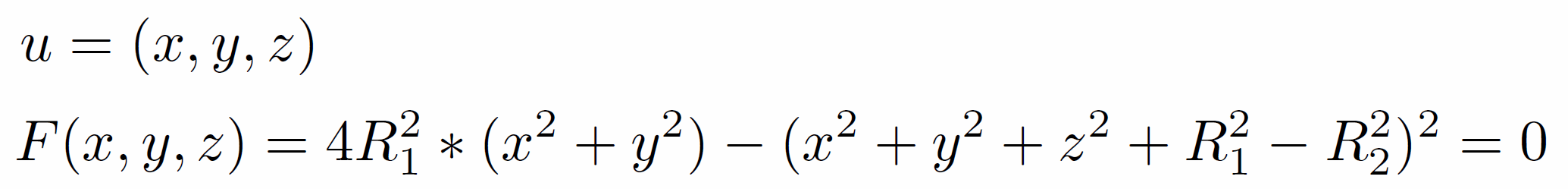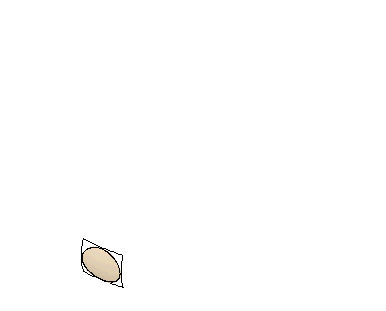The Torus is similar to the computation of the sphere.

### Cube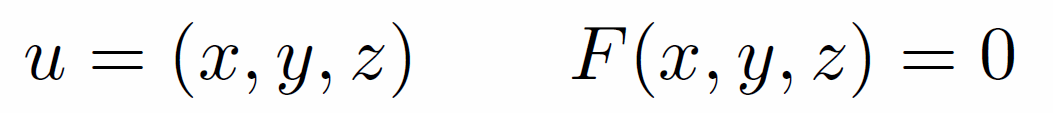Computing a 3-manifold embedded in 3-space: n=3, k=3

### (2,4) Mixed mode interaction in Taylor-Couette flow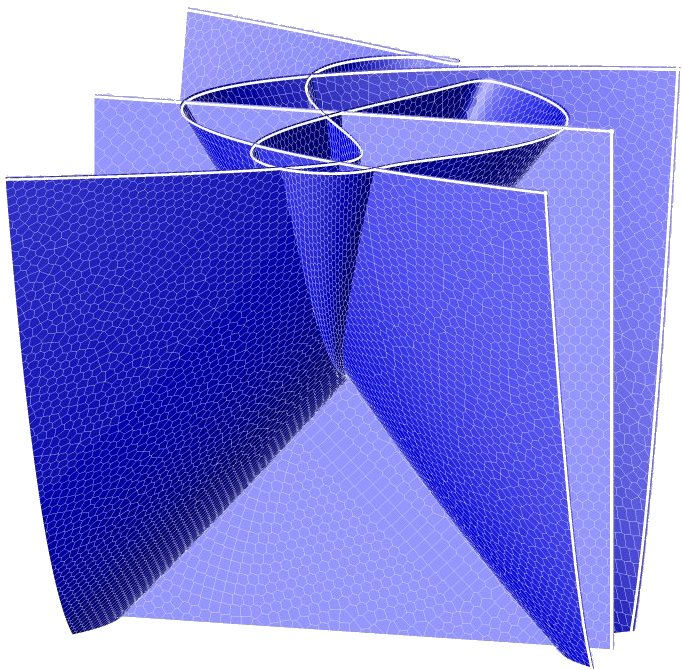### Complex Cusp Catastrophe